Courses

# Test: Solid Mechanics Level - 4

## 25 Questions MCQ Test Civil Engineering SSC JE (Technical) | Test: Solid Mechanics Level - 4

Description
This mock test of Test: Solid Mechanics Level - 4 for Civil Engineering (CE) helps you for every Civil Engineering (CE) entrance exam. This contains 25 Multiple Choice Questions for Civil Engineering (CE) Test: Solid Mechanics Level - 4 (mcq) to study with solutions a complete question bank. The solved questions answers in this Test: Solid Mechanics Level - 4 quiz give you a good mix of easy questions and tough questions. Civil Engineering (CE) students definitely take this Test: Solid Mechanics Level - 4 exercise for a better result in the exam. You can find other Test: Solid Mechanics Level - 4 extra questions, long questions & short questions for Civil Engineering (CE) on EduRev as well by searching above.
QUESTION: 1

Solution:
QUESTION: 2

Solution:
QUESTION: 3

### Principal strains at a point are + 100 × 10–6 and– 200 × 10–6. What is the maximum shear strain at the point ?

Solution: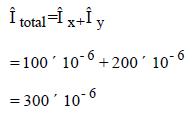QUESTION: 4

In a strained material one of the principal stresses is twice the other. The maximum shear stress in the same case ist max. Then, what is the value of the maximum principal stress ?

Solution:
QUESTION: 5

For a general two dimensional stress system, what are the coordinates of the centre of Mohr's circle?

Solution:
QUESTION: 6

The principal strains at a point in a body, under biaxial state of stress, are 1000 × 10–6 and –600×10–6. What is the maximum shear strain at that point ?

Solution: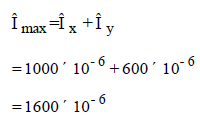QUESTION: 7

If the principal stresses and maximum shearing stresses are of equal numerical value at a point in a stressed body, the state of stress can be termed as

Solution:
QUESTION: 8

The state of plane stress at a point in a loaded member is given by:

sx = + 800 MPa

sy = + 200 MPa

txy = ± 400 MPa

The maximum principal stress and maximum shear stress are given by:

Solution:QUESTION: 9

Which of the following figures may represent Mohr's circle?

Solution:
QUESTION: 10

Auto frettage is the method of

Solution:
QUESTION: 11

From design point of view, spherical pressure vessels are preferred over cylindrical pressure vessels because they

Solution:
QUESTION: 12

In a thick cylinder pressurized from inside, the hoop stress is maximum at

Solution:
QUESTION: 13

A thin cylinder with closed lids is subjected to internal pressure and suported at the ends as shown in figure.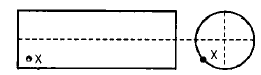The state of stress at point X is a s represented as

Solution:
QUESTION: 14

A thin cylinder with both ends closed is subjected to internal pressure p. The longitudinal stress at the surface has been calculated as so. maximum shear stress at the surface will be equal to

Solution:
QUESTION: 15

If a thick cylindrical shell is subjected to internal pressure. then hoop stress, radial stress and longitudinal stess at point in the thickness will be

Solution:
QUESTION: 16

The commonly used technique of strengthening thin pressure vessels is

Solution:
QUESTION: 17

Auto-frettage is a method of

Solution:
QUESTION: 18

Where does the maximum hoop stress in a thick cylinder under external pressure occur ?

Solution:
QUESTION: 19

A seamless pipe of diameter d m is to carry fluid under a pressure of P kN/cm2. The necessary
thickness t of metal in cm, if the maximum stress is not to exceeds kN/cm2 is

Solution:
QUESTION: 20

Constant bending moment over span 'l' will occur in

Solution:
QUESTION: 21

The bending moment (M) is constant over a length segment (I) of a beam the shearing force will also be constant over this length and is given by

Solution:
QUESTION: 22

The given figure shows a cantilever of span L subjected to a concentrated load P and a moment M at the free end. Deflection a the free end is given by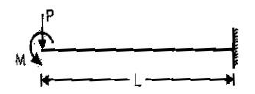Solution:
QUESTION: 23

For a cantilever beam of length 'L' flexural rigidity El ad loaded at its free end by a concentrated
load W, match List-I with List-II and select the correct answer using the codes below the lists: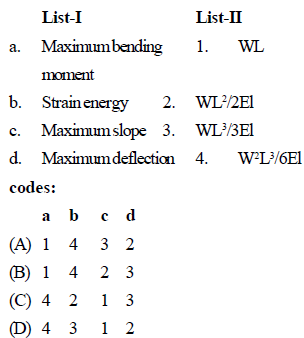Solution:
QUESTION: 24

A beam, built in both ends, carries a uniformly distributed load over its entire span as shown in
figure. Which one of the diagrams given below represents bending moment distribution along the length of the beam?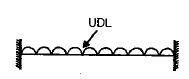Solution:
QUESTION: 25

A simply supported beam has equal overhanging lengths and carries equal concentrated loads P at ends. Bending moment over the length between the supports

Solution: